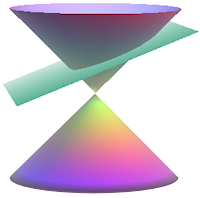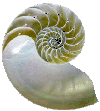Search IntMath
Close

450+ Math Lessons written by Math Professors and Teachers

5 Million+ Students Helped Each Year

1200+ Articles Written by Math Educators and Enthusiasts

Simplifying and Teaching Math for Over 23 Years

# Plane Analytical Geometry

By M Bourne### An interesting application from nature:

The Nautilus ShellSee: Equiangular spiral.

(Image from Tree of Life)

The curves that we learn about in this chapter are called conic sections. They arise naturally in many situations and are the result of slicing a cone at various angles.

Depending on where we slice our cone, and at what angle, we will either have a straight line, a circle, a parabola, an ellipse or a hyperbola. Of course, we could also get a single point, too.

## Why study analytic geometry?

Science and engineering involves the study of quantities that change relative to each other (for example, distance-time, velocity-time, population-time, force-distance, etc).

It is much easier to understand what is going on in these problems if we draw graphs showing the relationship between the quantities involved.

The study of calculus depends heavily on a clear understanding of functions, graphs, slopes of curves and shapes of curves. For example, in the Differentiation chapter we use graphs to demonstrate relationships between varying quantities.

## In this Chapter

We begin with the Distance Formula »

## Problem SolverThis tool combines the power of mathematical computation engine that excels at solving mathematical formulas with the power of GPT large language models to parse and generate natural language. This creates math problem solver thats more accurate than ChatGPT, more flexible than a calculator, and faster answers than a human tutor. Learn More.